Courses

# Sitting Arrangement MCQ 1

## 30 Questions MCQ Test Logical Reasoning for CLAT | Sitting Arrangement MCQ 1

Description
This mock test of Sitting Arrangement MCQ 1 for CLAT helps you for every CLAT entrance exam. This contains 30 Multiple Choice Questions for CLAT Sitting Arrangement MCQ 1 (mcq) to study with solutions a complete question bank. The solved questions answers in this Sitting Arrangement MCQ 1 quiz give you a good mix of easy questions and tough questions. CLAT students definitely take this Sitting Arrangement MCQ 1 exercise for a better result in the exam. You can find other Sitting Arrangement MCQ 1 extra questions, long questions & short questions for CLAT on EduRev as well by searching above.
QUESTION: 1

Solution:
QUESTION: 2

### Directions: Study the following information carefully to answer these questions: Eight friends J, K, L, M, N, O, P and Q are sitting around a circle facing the centre. J is not the neighbour of N. L is third to the right of K. Q is second to the left of N who is next to the right of L. O is not the neighbour of N or K and is to the immediate left of P.  Q. Which of the following groups has the first person sitting between the other two persons?

Solution:
• Eight friends J, K, L, M N, O, P and Q are sitting around a circle facing the center.
• J is not the neighbour of N.
• L is third to the right of K.
• Q is second to the left of N who is next to the right of L.
• O is not the neighbour of N or K and is to the immediate left of P.

So, the first person sitting between the other two persons is: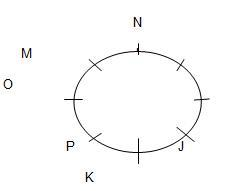QUESTION: 3

### Directions: Study the following and answer the questions given below: (i) Six persons L, K, M, N, O, and H are sitting in a circle and their faces are towards the center. (ii) K is between ‘O’ and ‘L’, and O is at the opposite side of H. (iii) N is to the left of ‘O’ ​Q. Who is sitting at the left of K?

Solution:
QUESTION: 4

Directions: Study the following and answer the questions given below:

(i) Five persons A, B, C, D, and E are sitting in a circle and their faces are towards the center.

(ii) D is between B and C.

(iii) B is to the left of E.

Q. ​Who is sitting between ‘A’ and ‘B’?

Solution:

The correct option is c.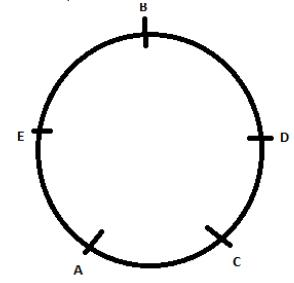QUESTION: 5

Directions: Study the following and answer the questions given below:

(i) Five persons A, B, C, D, and E are sitting in a circle and their faces are towards the center.

(ii) D is between B and C.

(iii) B is to the left of E.

Q. ​Who is to the right of ‘E’?

Solution:

In this circle ,
B is extreme left to E, E is extreme left to A, A is extreme left to C ,C is extreme left to D ,
as well as D is extreme left to B .
So , the 2nd line means that A is extreme right of E.
That's why,
A is the right answer.

QUESTION: 6

Directions: Study the following information and answer the questions given below:

M, N, P, R, T, W, F, and H are sitting around a circle facing the center. P is third to the left of M and second to the right of T. N is second to the right of P. R is second to the right of  W, who is second to the right of M. F is not an immediate neighbour of the P.

Q. Who is to the immediate right of P?

Solution:
QUESTION: 7

Directions: Study the following information carefully and answer the questions. A, B, C, D, E, F, G, and H are sitting around a circle, facing the center. A sits fourth to the right of H while second to the left of F. C is not the neighbor of F and B. D sits third to the right of C. H never sits next to G.

Q. Who amongst the following sits between B and D?

Solution:

The sequence which follows is as - A, G, C, E, H, B, F, & D
Put the letters in a circle, so F will sit between B and D.

QUESTION: 8

Directions: Study the following information and answer the questions given below:

M, N, P, R, T, W, F, and H are sitting around a circle facing the centre. P is third to the left of M and second to the right of T. N is second to the right of P. R is second to the right of W, who is second to the right of M. F is not an immediate neighbour of the P.

Q. Who is to the immediate left of R?

Solution:
QUESTION: 9

Directions: Study the following information and answer the questions given below:

M, N, P, R, T, W, F and H are sitting around a circle facing the center. P is third to the left of M and second to the right of T. N is second to the right of P. R is second to the right of  W, who is second to the right of M. F is not an immediate neighbour of the P.

Q. Who is to third to the right of H?

Solution:
QUESTION: 10

Directions: Study the following information and answer the questions given below:

M, N, P, R, T, W, F, and H are sitting around a circle facing the centre. P is third to the left of M and second to the right of T. N is second to the right of P. R is second to the right of  W, who is second to the right of M. F is not an immediate neighbour of the P.

Q. Who is second to the right of F?

Solution:
QUESTION: 11

​Directions: Study the following information and answer the questions given below:

M, N, P, R, T, W, F and H are sitting around a circle facing the centre. P is third to the left of M and second to the right of T. N is second to the right of P. R is second to the right of  W, who is second to the right of M. F is not an immediate neighbour of P.

Q. In which of the following is the first person sitting in between the second and the third person?

Solution:
QUESTION: 12

Directions :

(i) A, B, C, D, E, F, G AND H are sitting along a circle facing the centre.

(ii) D is the neighbour of A but not of H.

(iii) B is the neighbour of F and 4th to the left of D.

(iv) E is the neighbour of H and 3rd to the right of F.

(v) C is neither the neighbour of A nor of G.

Q. Which of the following is correct?

Solution:
QUESTION: 13

Directions :

(i) A, B, C, D, E, F, G AND H are sitting along a circle facing the centre.

(ii) D is the neighbour of A but not of H.

(iii) B is the neighbour of F and 4th to the left of D.

(iv) E is the neighbour of H and 3rd to the right of F.

(v) C is neither the neighbour of A nor of G.

Q. Which of the following is wrong?

Solution:

The correct option is C.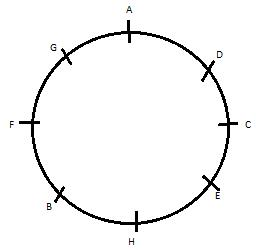QUESTION: 14

Directions :

(i) A, B, C, D, E, F, G AND H are sitting along a circle facing the centre.

(ii) D is the neighbour of A but not of H.

(iii) B is the neighbour of F and 4th to the left of D.

(iv) E is the neighbour of H and 3rd to the right of F.

(v) C is neither the neighbour of A nor of G.

Q. Which of the following groups has the 2nd person sitting between the 1st and the 3rd?

Solution: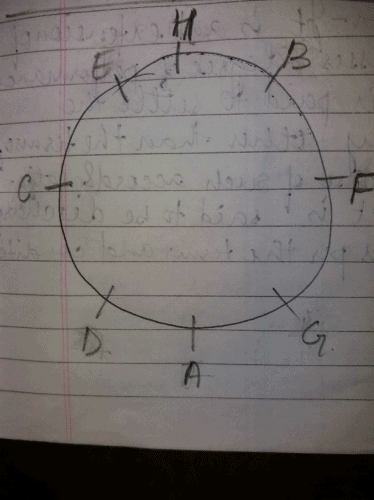QUESTION: 15

Directions :

A, B, C, D, E, F, G and H are sitting around a circle facing the centre. D is fourth to the right of H and second to the left of B. F is fourth to the right of B. C is fourth to the right of E who is not an immediate neighbour of B or D. A is not an immediate neighbour of D.

Q. What is B’s position with respect to G?

Solution: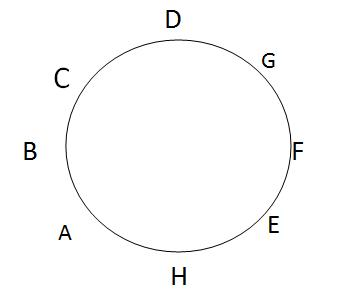B is third to the right of G.

QUESTION: 16

Directions :

(i) A, B, C, D, E, F, G AND H are sitting along a circle facing the centre.

(ii) D is the neighbour of A but not of H.

(iii) B is the neighbour of F and 4th to the left of D.

(iv) E is the neighbour of H and 3rd to the right of F.

(v) C is neither the neighbour of A nor of G.

Q. Which of the following pairs has three persons sitting between them?

Solution:
QUESTION: 17

Directions :

(i) A, B, C, D, E, F, G AND H are sitting along a circle facing the centre.

(ii) D is the neighbour of A but not of H.

(iii) B is the neighbour of F and 4th to the left of D.

(iv) E is the neighbour of H and 3rd to the right of F.

(v) C is neither the neighbour of A nor of G.

Q. If C and G interchange their positions, which of the following will indicate A’s position?

Solution:
QUESTION: 18

Directions: Study the following and answer the questions given below:

(i) Six persons Raj, Ram, Rajesh, Ramesh, Rohit, and Ravi are sitting in a circle and their faces are towards the circle.

(ii) Ravi is to the left of Ram and is the opposite of Ramesh.

(iii) Rajesh is to the right of Rohit.

Q. Who is sitting between Rohit and Raj?

Solution:
QUESTION: 19

Directions: Study the following and answer the questions given below:

(i) Six persons Raj, Ram, Rajesh, Ramesh, Rohit, and Ravi are sitting in a circle and their faces are towards the circle.

(ii) Ravi is to the left of Ram and is the opposite of Ramesh.

(iii) Rajesh is to the right of Rohit.

Q. Who is to the left of Ramesh?

Solution:

The correct option is B.

It is clear from the given image.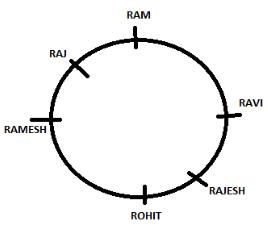QUESTION: 20

Directions: Study the following information carefully and answer the questions given below:
B, M, K, H, T, R, D, W and A are sitting around a circle facing at the centre. R is third to the right of B. H is second to the right of A who is second to the right of R. K is third to the right of T who is not an immediate neighbour of H. D is second to the left of T. M is fourth to the right of W.

​Q. Who is to the immediate left of W?

Solution: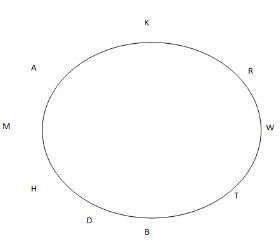QUESTION: 21

Directions: Study the following information carefully and answer the questions given below:
B, M, K, H, T, R, D, W and A are sitting around a circle facing at the centre. R is third to the right of B. H is second to the right of A who is second to the right of R. K is third to the right of T who is not an immediate neighbour of H. D is second to the left of T. M is fourth to the right of W.

Q. Who is third to the left of M?

Solution:

The correct option is D.

Explanation

T is not immediate neighbor of H. D is second to the left of T and K is third to the right of T. so T can sit in any of the position left vacant between B and R but if T is the neighbor of R then D cannot sit second to the left of T. so only one position left for T that is immediate right of B.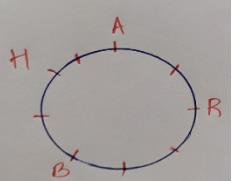Now here we left with two vacant positions which are for M and  W, M is fourth to the right of W. Means that W is in between R and T and M is between A and H.

From the given information the arrangements can be as follows.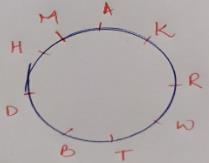QUESTION: 22

Directions: Study the following information carefully and answer the questions given below:
B, M, K, H, T, R, D, W and A are sitting around a circle facing at the centre. R is third to the right of B. H is second to the right of A who is second to the right of R. K is third to the right of T who is not an immediate neighbour of H. D is second to the left of T. M is fourth to the right of W.

​Q. Who is third to the left of H?

Solution:
QUESTION: 23

Directions: Study the following information carefully and answer the questions given below:
B, M, K, H, T, R, D, W and A are sitting around a circle facing at the centre. R is third to the right of B. H is second to the right of A who is second to the right of R. K is third to the right of T who is not an immediate neighbour of H. D is second to the left of T. M is fourth to the right of W.

​Q. Who is to the immediate left of D?

Solution:
QUESTION: 24

Directions: Study the following information carefully and answer the questions given below:
B, M, K, H, T, R, D, W and A are sitting around a circle facing at the centre. R is third to the right of B. H is second to the right of A who is second to the right of R. K is third to the right of T who is not an immediate neighbour of H. D is second to the left of T. M is fourth to the right of W.

​Q. In which of the following combinations is the third person sitting in between the first and the second persons?

Solution: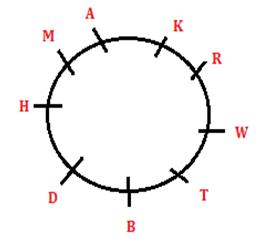So R is between W and K

WKR is the correct answer.

QUESTION: 25

Directions: On the basis of the information given below, carefully answer the questions:

(i) P, Q, R, S, and T are sitting in a circle facing the center.

(ii) R is the immediate left of T.

(iii) P is between S and T.

Q. Who is to the immediate left of R?

Solution:
QUESTION: 26

Directions: On the basis of the information given below, carefully answer the questions:

P, Q, R, S, T, U, and V are sitting around a circular table facing the center

R is next to the left of U and V is second to the left of R.

P is sitting third to the left of T.

Q is between S and T.

Q. Which of the following is false?

Solution:
QUESTION: 27

Six friends A, B, C, D, E and F are sitting in a closed circle facing the centre. A is facing D.C is between A and B.F is between E and A. Who is to the immediate left of B?

Solution:

QUESTION: 28

Directions: Read the following information carefully and answer the questions given below it:

A, B, C, D, E, and F are seated in a circle facing the center. D is between F and B. A is second to the left of D and second to the right of E.

Q. Who is facing A?

Solution:

The correct option is D.

Here direction of F and B in respect to D is not given.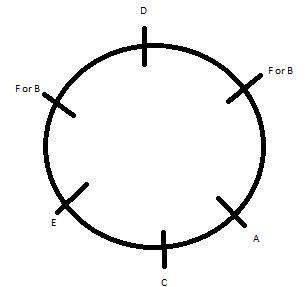QUESTION: 29

Directions: Read the following information carefully and answer the questions given below it:

A, B, C, D, E, and F are seated in a circle facing the center. D is between F and B. A is second to the left of D and second to the right of E.

Q. Who among following is facing D?

Solution:
QUESTION: 30

Directions: These questions are based on the following information:

Eight persons L, M, N, P, Q, R, S and T sitting around a circular table facing the centre. Q is not the neighbour of P or R. M is second to the left of T and third to the right of P. R is third to the left of N, who is to the immediate left of T. L is second to the right of P.

​Q. Which of the following pairs of persons represents the neighbours of T?

Solution: